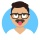Home
IT Knowledge
Inspiration
Languages
EN

# Python - replace first n characters in string

0 points
Created by:Zachary
316

In this article, we would like to show you how to replace first n characters in string in Python.

Quick solution:

``````text = "ABCD ABCD ABCD"
n = 3
replacement = "XYZ"
result = text.replace(text[0:n], replacement, 1)

print(result)  # XYZD ABCD ABCD``````

## Practical example using `replace()` method

In this example, we use `replace()` method to replace first `n` characters in the `text` string.

We use the following syntax:

``replace(old, new, count)``

Where:

• `old` - is a substring to replace,
• `new` - is the replacement,
• `count` (optional argument) - is the number of times we want to replace the specified substring. You need to set it to `1`, so you won't replace anything but the first occurrence of the substring.

Practical example:

``````text = "ABCD ABCD ABCD"
n = 3
replacement = "XYZ"
result = text.replace(text[0:n], replacement, 1)

print("Before: ", text)    # ABCD ABCD ABCD
print("After:  ", result)  # XYZD ABCD ABCD``````

Output:

``````Before:  ABCD ABCD ABCD
After:   XYZD ABCD ABCD``````

Note:

If you don't set the `count` argument, by default the `replace()` method will replace all occurrences of the `ABC` substring.

Output:

``````Before:  ABCD ABCD ABCD
After:   XYZD XYZD XYZD``````